Ex 10.4

Chapter 10 Class 12 Vector Algebra
Serial order wise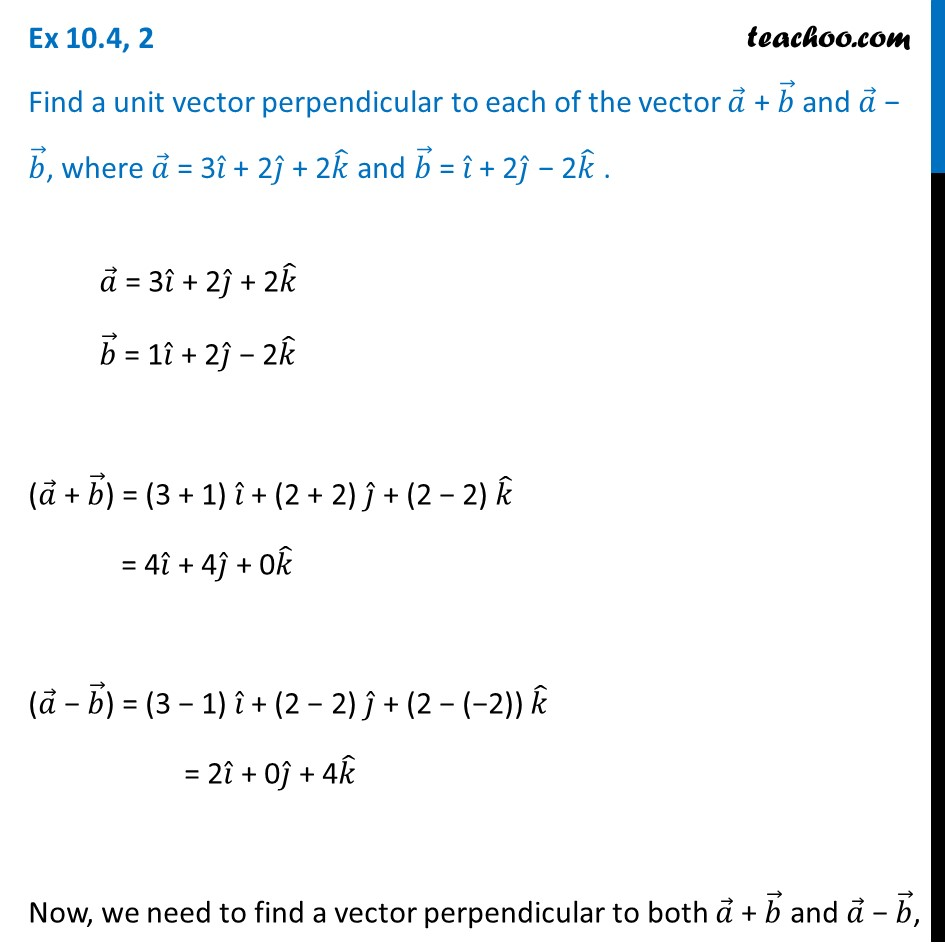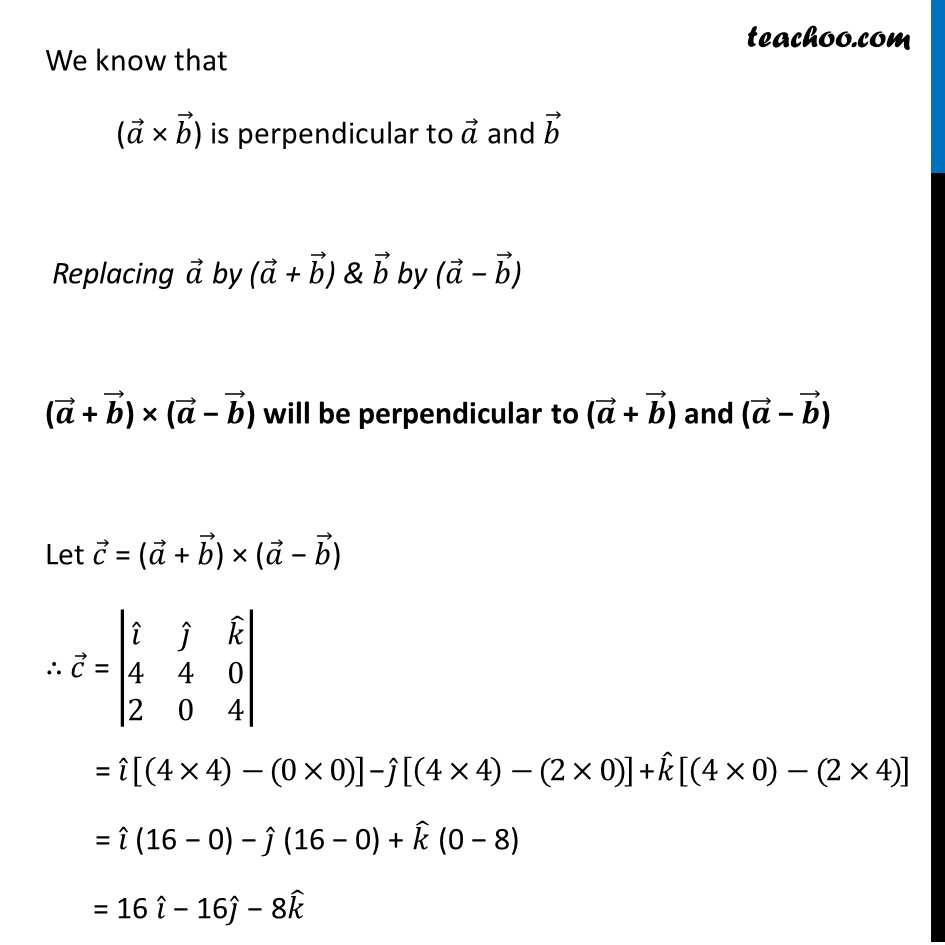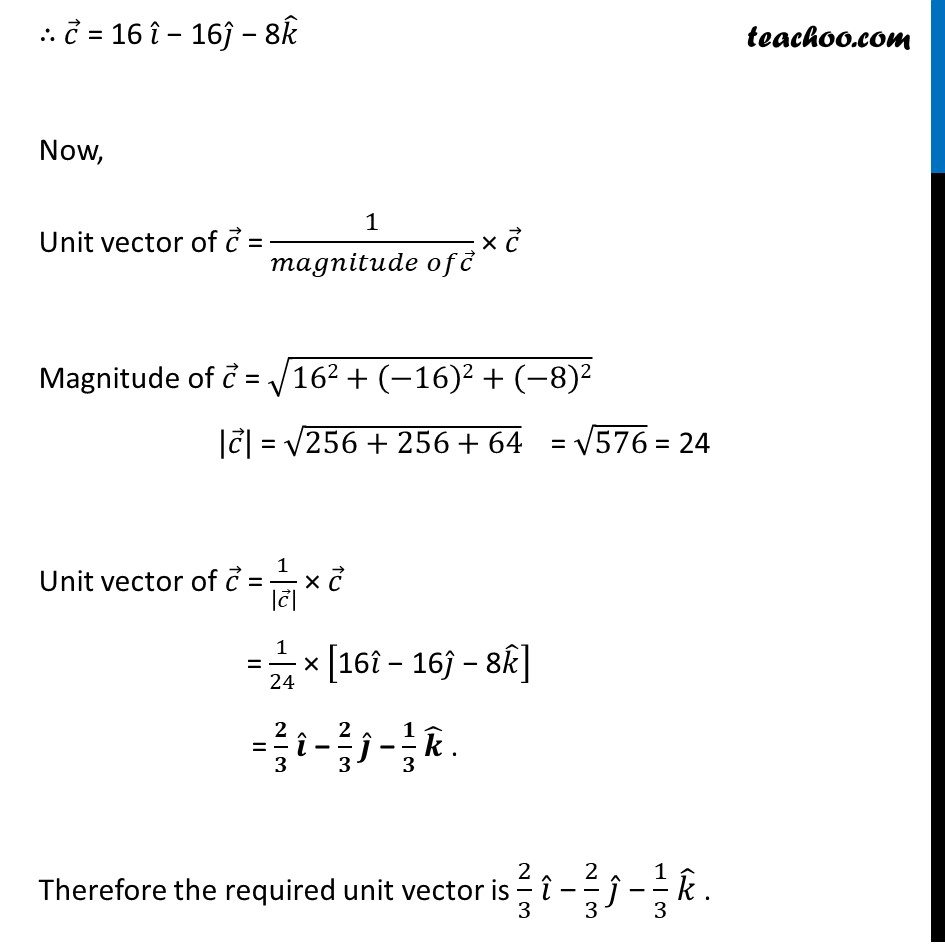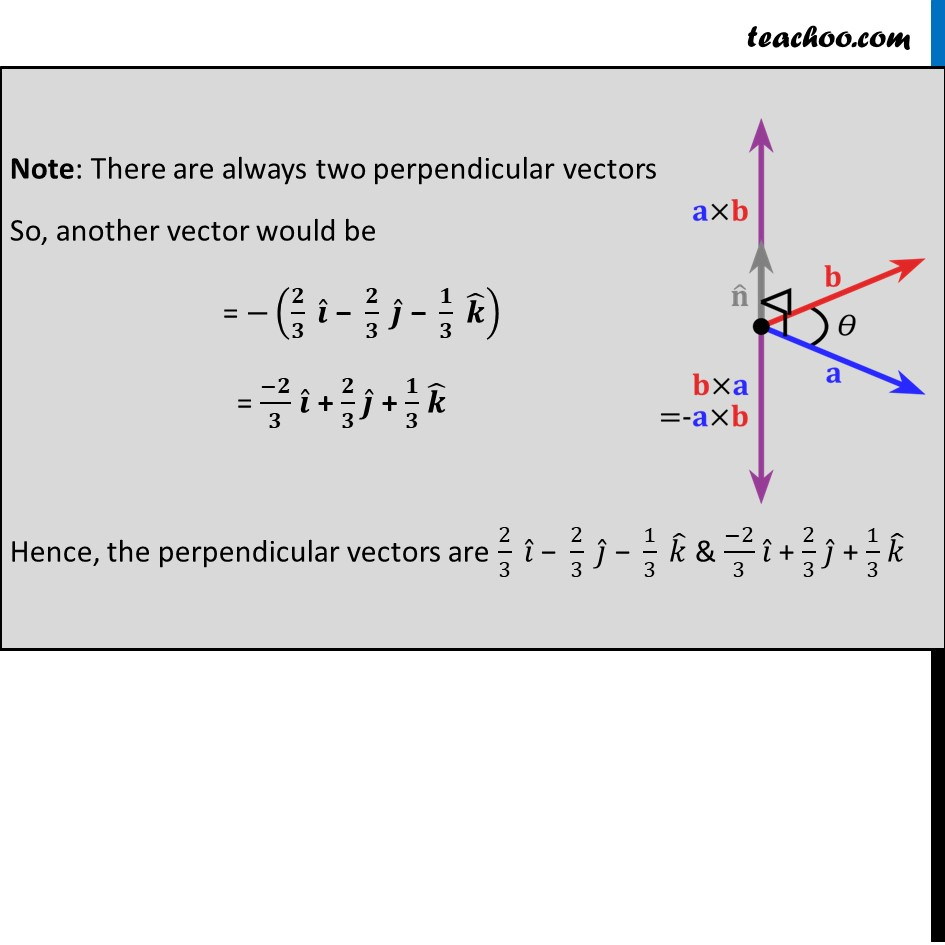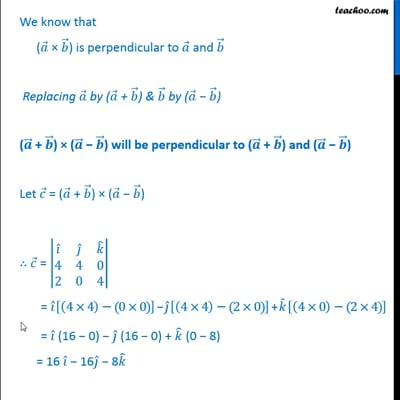This video is only available for Teachoo black users

Introducing your new favourite teacher - Teachoo Black, at only ₹83 per month

### Transcript

Ex 10.4, 2 Find a unit vector perpendicular to each of the vector 𝑎 ⃗ + 𝑏 ⃗ and 𝑎 ⃗ − 𝑏 ⃗, where 𝑎 ⃗ = 3𝑖 ̂ + 2𝑗 ̂ + 2𝑘 ̂ and 𝑏 ⃗ = 𝑖 ̂ + 2𝑗 ̂ − 2𝑘 ̂ .𝑎 ⃗ = 3𝑖 ̂ + 2𝑗 ̂ + 2𝑘 ̂ 𝑏 ⃗ = 1𝑖 ̂ + 2𝑗 ̂ − 2𝑘 ̂ (𝑎 ⃗ + 𝑏 ⃗) = (3 + 1) 𝑖 ̂ + (2 + 2) 𝑗 ̂ + (2 − 2) 𝑘 ̂ = 4𝑖 ̂ + 4𝑗 ̂ + 0𝑘 ̂ (𝑎 ⃗ − 𝑏 ⃗) = (3 − 1) 𝑖 ̂ + (2 − 2) 𝑗 ̂ + (2 − (−2)) 𝑘 ̂ = 2𝑖 ̂ + 0𝑗 ̂ + 4𝑘 ̂ Now, we need to find a vector perpendicular to both 𝑎 ⃗ + 𝑏 ⃗ and 𝑎 ⃗ − 𝑏 ⃗, We know that (𝑎 ⃗ × 𝑏 ⃗) is perpendicular to 𝑎 ⃗ and 𝑏 ⃗ Replacing 𝑎 ⃗ by (𝑎 ⃗ + 𝑏 ⃗) & 𝑏 ⃗ by (𝑎 ⃗ − 𝑏 ⃗) (𝒂 ⃗ + 𝒃 ⃗) × (𝒂 ⃗ − 𝒃 ⃗) will be perpendicular to (𝒂 ⃗ + 𝒃 ⃗) and (𝒂 ⃗ − 𝒃 ⃗) Let 𝑐 ⃗ = (𝑎 ⃗ + 𝑏 ⃗) × (𝑎 ⃗ − 𝑏 ⃗) ∴ 𝑐 ⃗ = |■8(𝑖 ̂&𝑗 ̂&𝑘 ̂@█(4@2)&█(4@0)&█(0@4))| = 𝑖 ̂ [(4×4)−(0×0)] − 𝑗 ̂ [(4×4)−(2×0)] + 𝑘 ̂ [(4×0)−(2×4)] = 𝑖 ̂ (16 − 0) − 𝑗 ̂ (16 − 0) + 𝑘 ̂ (0 − 8) = 16 𝑖 ̂ − 16𝑗 ̂ − 8𝑘 ̂ ∴ 𝑐 ⃗ = 16 𝑖 ̂ − 16𝑗 ̂ − 8𝑘 ̂ Now, Unit vector of 𝑐 ⃗ = 1/(𝑚𝑎𝑔𝑛𝑖𝑡𝑢𝑑𝑒 𝑜𝑓𝑐 ⃗ ) × 𝑐 ⃗ Magnitude of 𝑐 ⃗ = √(162+(−16)2+(−8)2) |𝑐 ⃗ | = √(256+256+64) = √576 = 24 Unit vector of 𝑐 ⃗ = 1/|𝑐 ⃗ | × 𝑐 ⃗ = 1/24 × ["16" 𝑖 ̂" − 16" 𝑗 ̂" − 8" 𝑘 ̂ ] = 𝟐/𝟑 𝒊 ̂ − 𝟐/𝟑 𝒋 ̂ − 𝟏/𝟑 𝒌 ̂ . Therefore the required unit vector is 2/3 𝑖 ̂ − 2/3 𝑗 ̂ − 1/3 𝑘 ̂ . Note: There are always two perpendicular vectors So, another vector would be = −(𝟐/𝟑 " " 𝒊 ̂" − " 𝟐/𝟑 " " 𝒋 ̂" − " 𝟏/𝟑 " " 𝒌 ̂ ) = (−𝟐)/𝟑 𝒊 ̂ + 𝟐/𝟑 𝒋 ̂ + 𝟏/𝟑 𝒌 ̂ Hence, the perpendicular vectors are 2/3 " " 𝑖 ̂" − " 2/3 " " 𝑗 ̂" − " 1/3 " " 𝑘 ̂ & (−2)/3 𝑖 ̂ + 2/3 𝑗 ̂ + 1/3 𝑘 ̂